Video Tutorial Entropy

Quick Notes Entropy

• Entropy, S, is a measure of disorder or 'the dispersal of energy' within a system, with the units JK-1mol -1.
• If there is a high level disorder - it has a high entropy.
• If there is a lower level disorder - it has a lower entropy.
• Solids have low entropies; gases have high entropies. Going from solid to gas increases the entropy of a system as the level of disorder increases.
•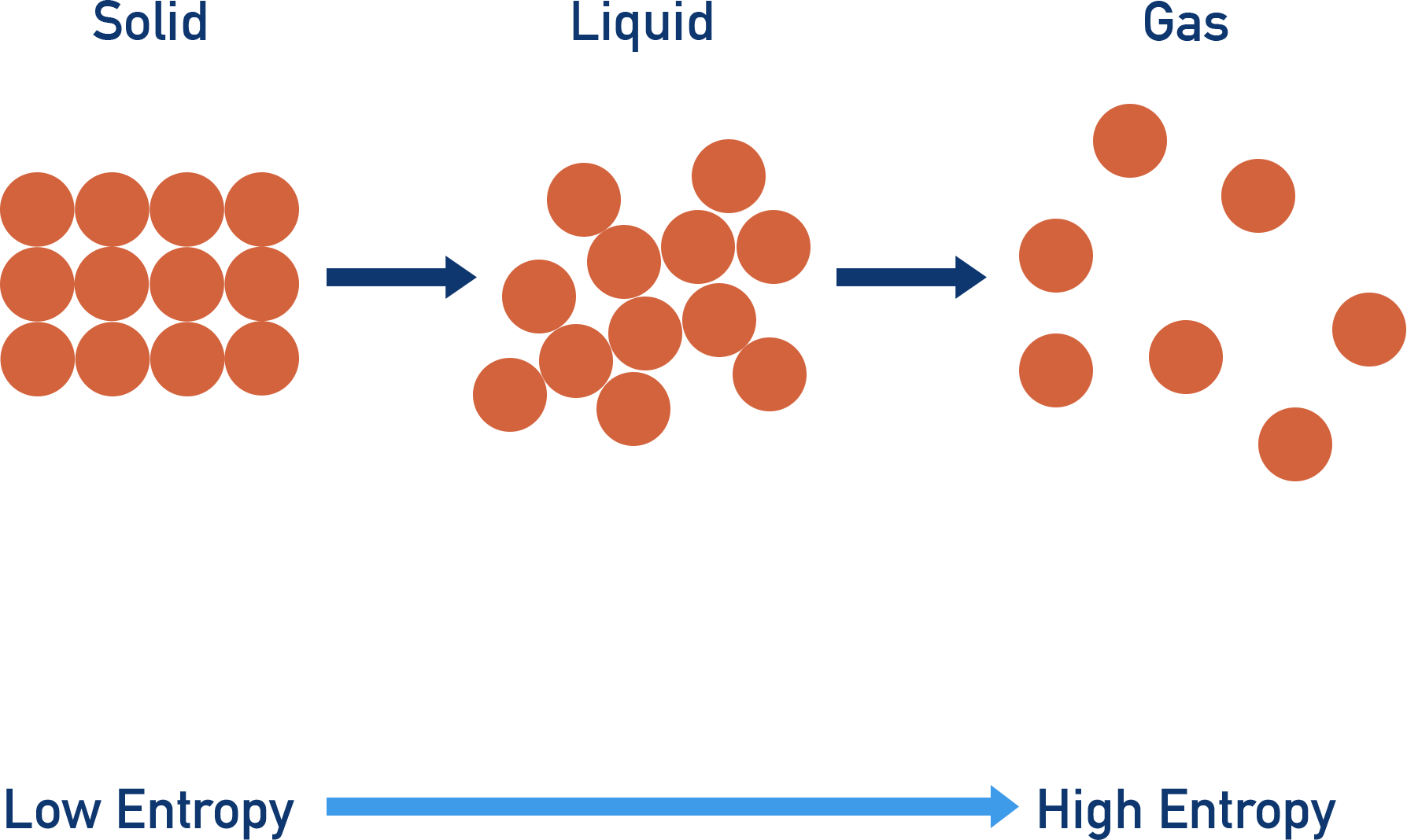• Entropy increases with temperature - the higher the temperature of a system, the greater its entropy.

• All systems have internal energy (enthalpy, H), the entropy content of a system refers to the proportion of this energy that is unable to flow out as heat - it is unavailable or 'dispersed' energy.
• Only a group of particles (a system) can have 'entropy', one particle has no associated entropy.
• The greater the number of ways energy can be distributed amongst particles, the more dispersed it becomes. Less of it is available to flow out as heat, meaning higher entropy content.
• If there are lots of possible of ways of distributing the energy amongst particles in a system, there is high disorder and it has a high entropy.
• If there are fewer possible ways of distributing the energy amongst particles in a system, there is less disorder and it has a lower entropy.

Full Notes Entropy

Entropy is pretty strange when you come accross it for the first time!

This page has been split into two sections - the first is a simplified and general outline for entropy as it is likely to be examined at this level, the second is a bit more in-depth but will help when tackling things like total entropy and gibbs free energy.

## What is Entropy? (simpler)

Entropy refers to the amount of energy a system has that can't flow out of it as heat, it is unavailable or 'dispersed' energy. The units are J K-1mol-1 (Joules per Kelvin per mole).

The more possible ways that particles can be arranged in a system, the higher its entropy. This is why entropy is sometimes described as a 'measure of disorder'. Disorder here means the possible ways particles can be arranged, see below for a more advanced description.

Obviously the more particles you have in a system, the greater the number of ways you can arrange them all, giving a higher level of disorder. As a result, a system with more particles in has a higher entropy than a similar system with fewer particles in. This is why entropy has moles (per mol) in its units. But it isn’t just the number of particles that has an impact on the potential disorder of a system. The state of a system (solid, liquid or gas) also affects its entropy.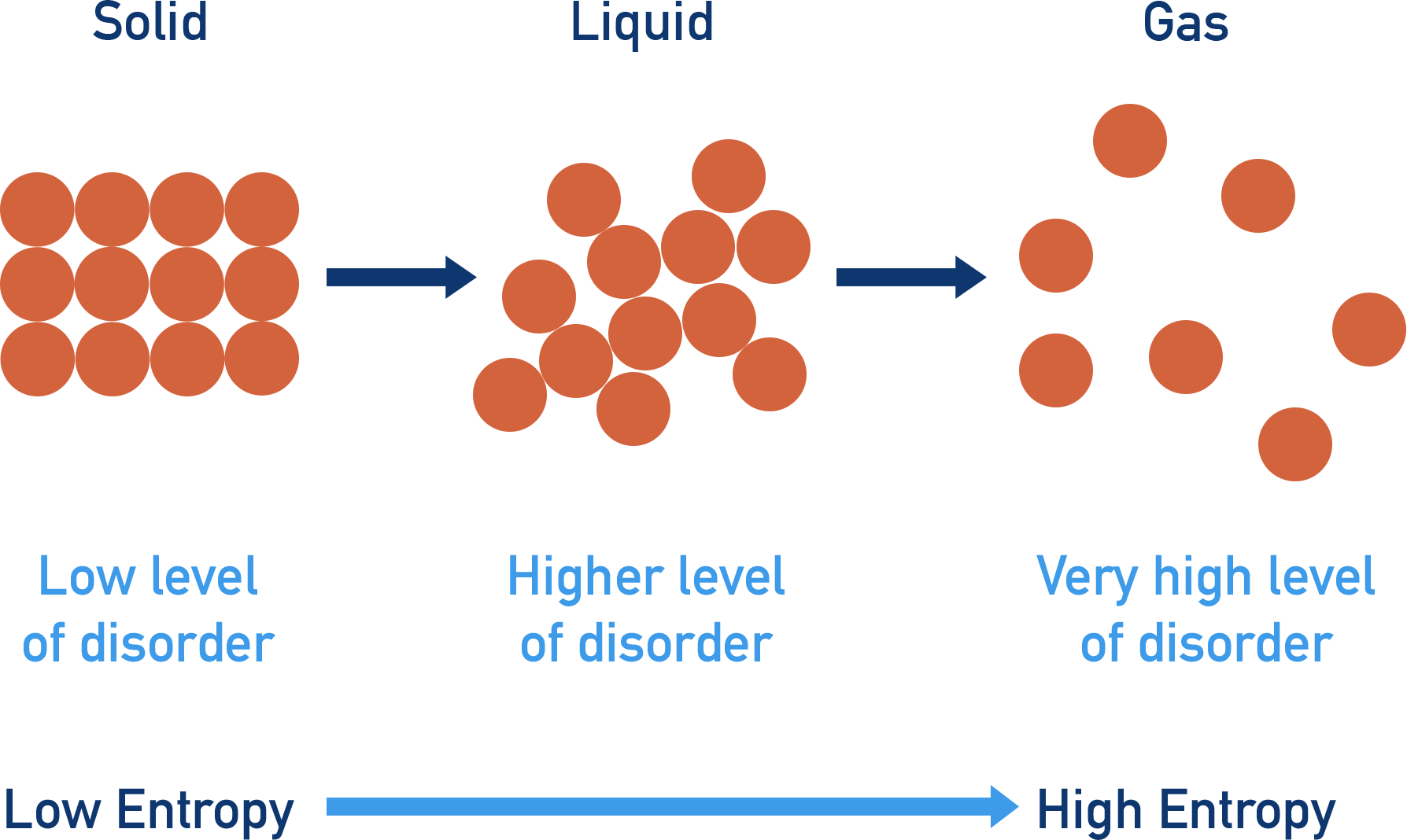There are a limited number of ways to arrange particles in solids, meaning they have a low level of disorder and a low entropy. In a liquid, there are lots of possible ways the particles can be arranged, meaning a higher level of disorder than a solid and a higher entropy. In gases, there is a huge level of disorder, giving a very high level of entropy compared to solids and liquids.

The level of disorder in a system also increases with temperature. This means entropy increases with temperature. This is why entropy has temperature (per Kelvin, K) in its units.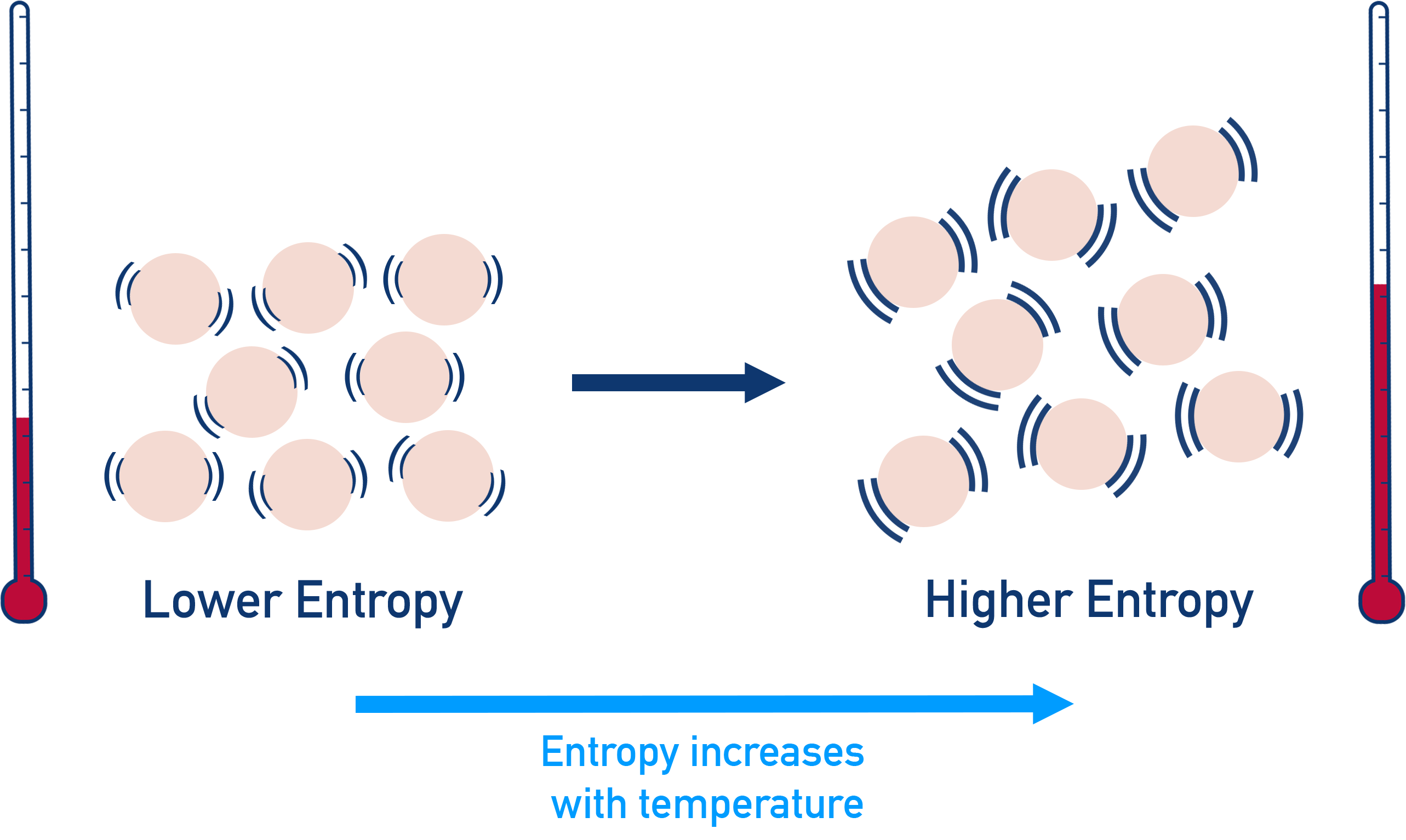For reasons explained below, the entropy of a system is linked to stability and energy, meaning it is measured with Joules (the unit for energy). It is represented with the letter S. Always remember the unit of energy in entropy is Joules, unlike enthalpy where the unit of energy used is kJ.

To summarise:

For a system...

more particles = higher entropy, fewer particles = lower entropy

higher temperature = higher entropy, lower temperature = lower entropy

gas = higher entropy, solid = lower entropy

## What actually is Entropy? (more advanced)

All substances and systems have internal energy (enthalpy, H). The only way any of this energy can theoretically 'leave' a system is in the form of heat, this is why enthalpy is referred to as 'heat content' (see Enthalpy).

As energy gets spread out amongst a group of particles however, some of it becomes unavailable to flow out as heat. This proportion of energy is referred to as 'entropy' or dispersed energy. We can think of the enthalpy of a system as being made up of 'useful' energy that can flow out as heat and entropy, 'unavailable' energy that can't flow out of the system as heat.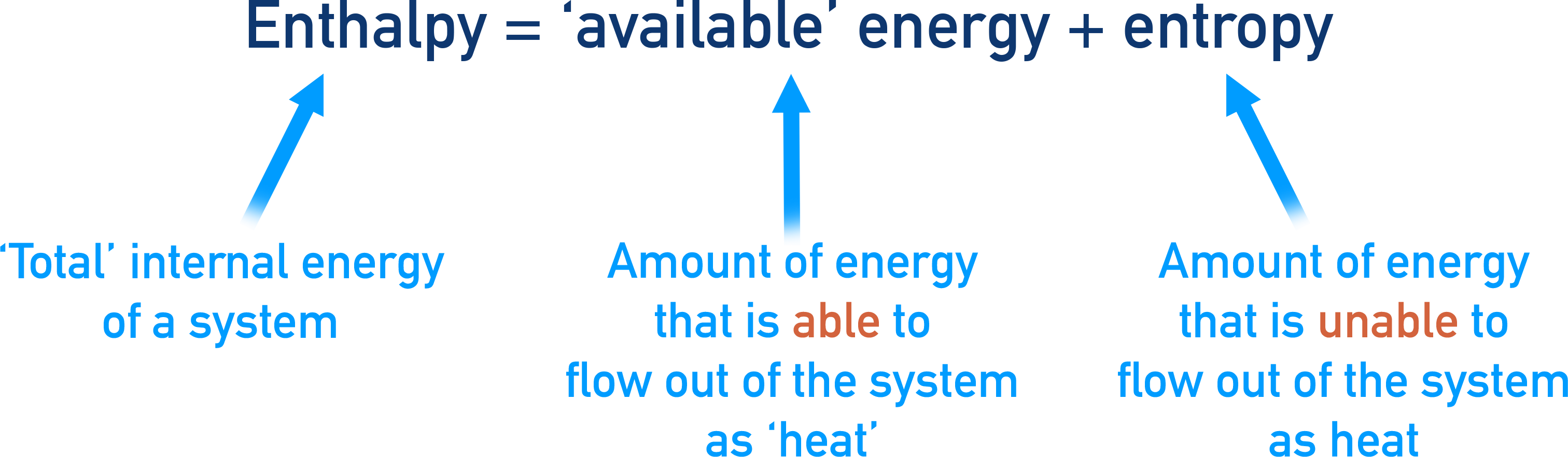All particles have ‘energy’ and this energy is made up of lots of small bits of energy, that can't be broken down into smaller amounts.

The particles in a system are constantly sharing these ‘bits’ of energy between them, meaning the particles in a system can all have different amounts of energy at any one time.Heat can flow out of a system because of this - the more 'unevenly' energy is distributed amongst particles, the easier it is for heat to leave a system (lower entropy). Equally, if energy is more evenly distributed, it becomes less able flow out of the system as heat (higher entropy).

The more possible ways there are to distribute energy amongst particles, the more likely it is that the energy will be ‘evenly’ spread or distributed amongst them all.

This is why entropy is linked to potential disorder: The greater the potential disorder, the more possible ways of distributing the energy amongst particles, meaning a more even spread of energy is likely, leaving less energy available to flow out as heat.

## Factors that affect entropy (more advanced)

As the number of particles in a system increases, there are more possible ways (increased disorder) the energy can be distributed amongst them all - meaning it is more likely to be evenly spread out.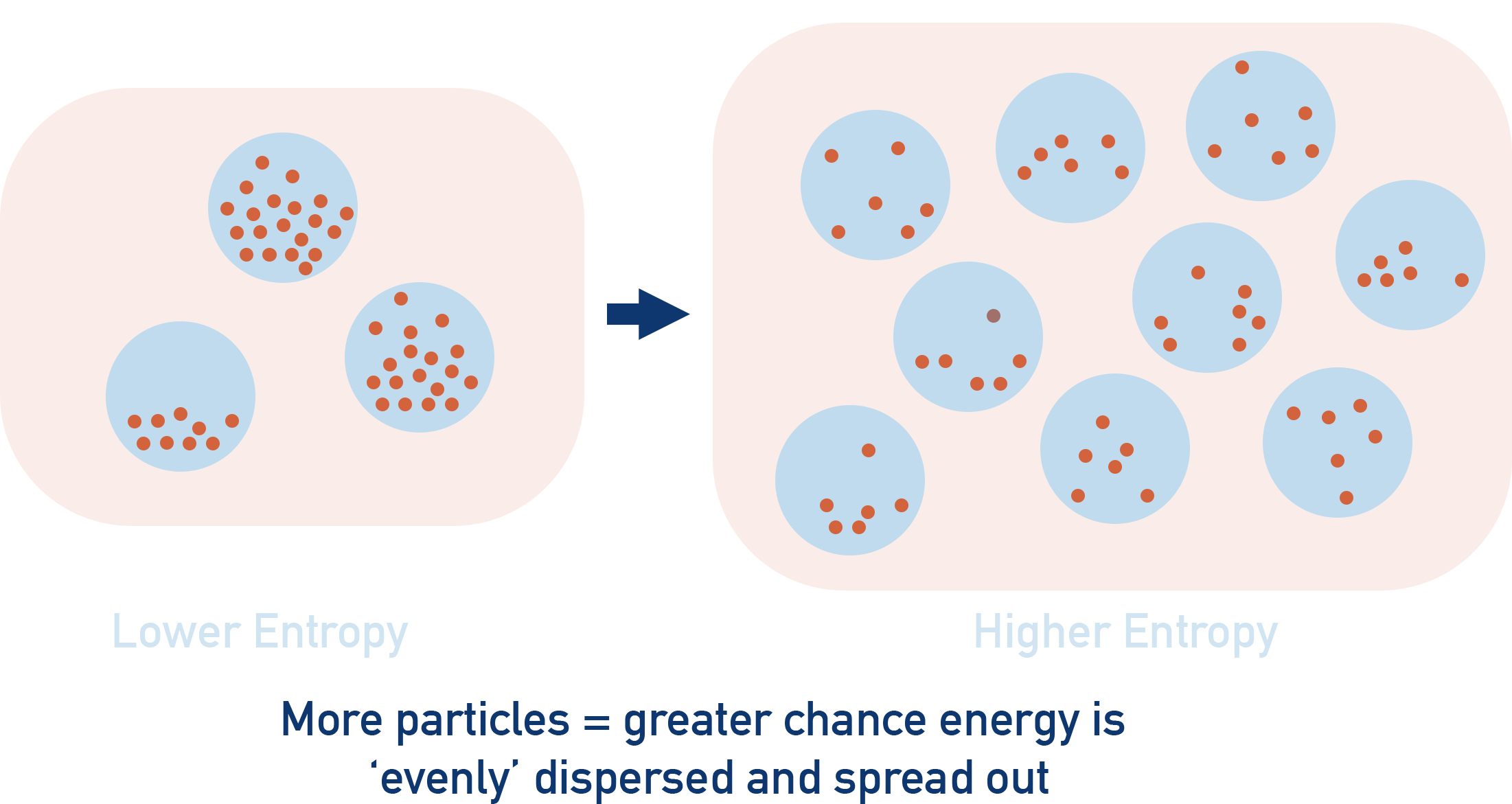This is why entropy is higher for systems with more particles in, there are more possible ways to distribute the energy amongst the particles. It is more likely the energy will be evenly spread out (dispersed) and less energy would be available to flow out of the system as heat.

As temperature increases, there is more energy amongst particles - meaning more possible ways to distribute or arrange the energy. This gives a greater chance that the energy will be evenly ‘spread out’ (entropy). Because of this, the entropy of a system is based on its temperature, meaning kelvin has to be included in its units.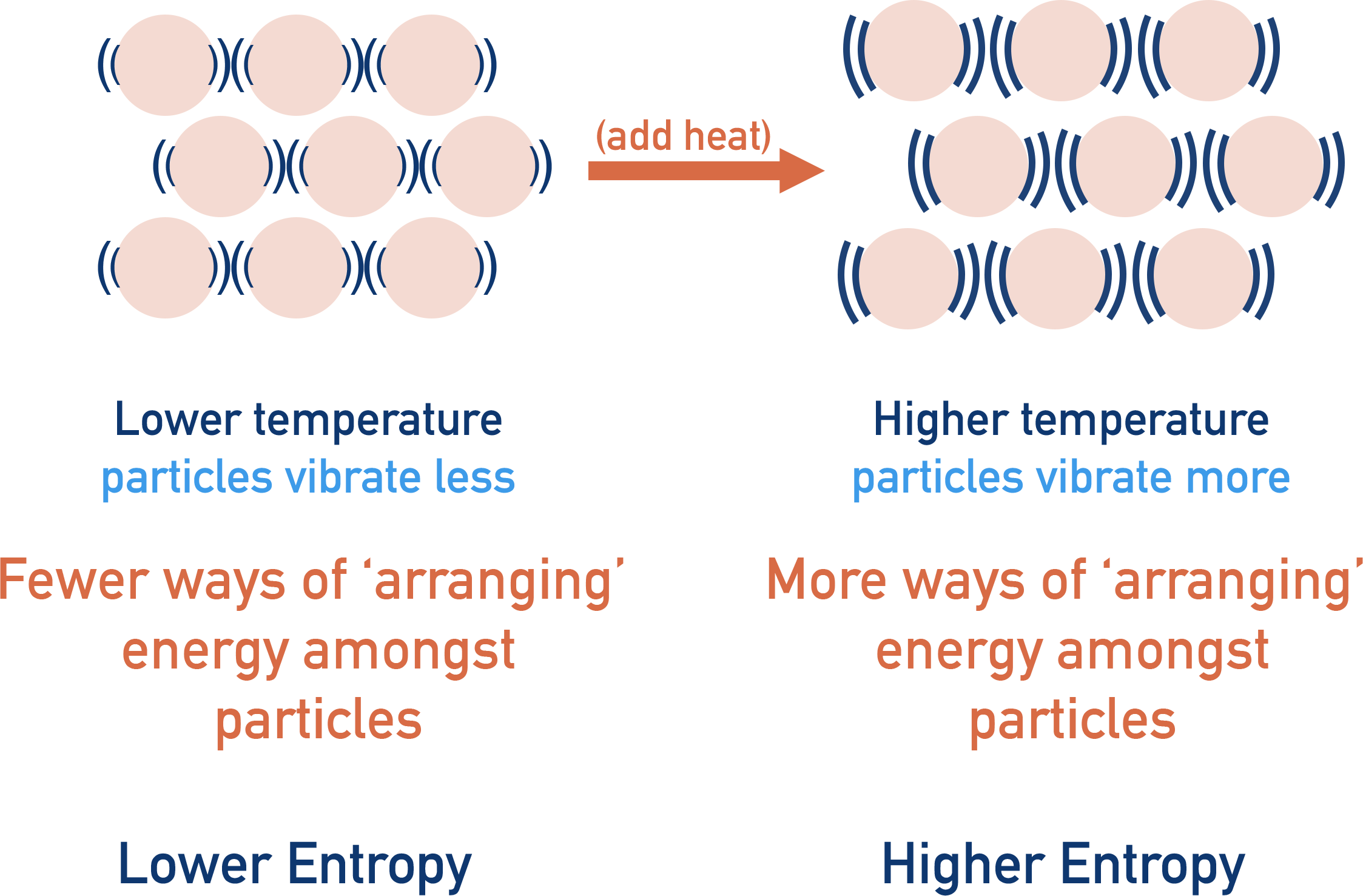So as the potential arrangements of energy amongst particles or ‘disorder’ in a system increases, entropy also increases - explaining why solids have a lower entropy than liquids and gases (see above). But it isn’t actually the particles we are interested in, it is how the energy the particles have gets shared or distributed between them all.

Entropy is the proportion of energy that a system has, at a given temperature, that can't flow out of it as heat. It is ‘unavailable’ energy.

It is based on the probability of energy distribution amongst particles in a system - the more possible ways energy can be distributed (potential disorder), the more likely the energy will be evenly distributed.

The higher the entropy content of a system, the more ‘stable’ it is. Energy is spread out more. In order for any reaction or process to occur, stability of the universe has to increase and the entropy of the universe must increase during a reaction or process.

Be careful though - the entropy of an individual system doesn’t have to increase, only the entropy of the whole universe. For example, you can take a gas system that has a high entropy and put it under high pressure to force it to become a liquid - lower entropy.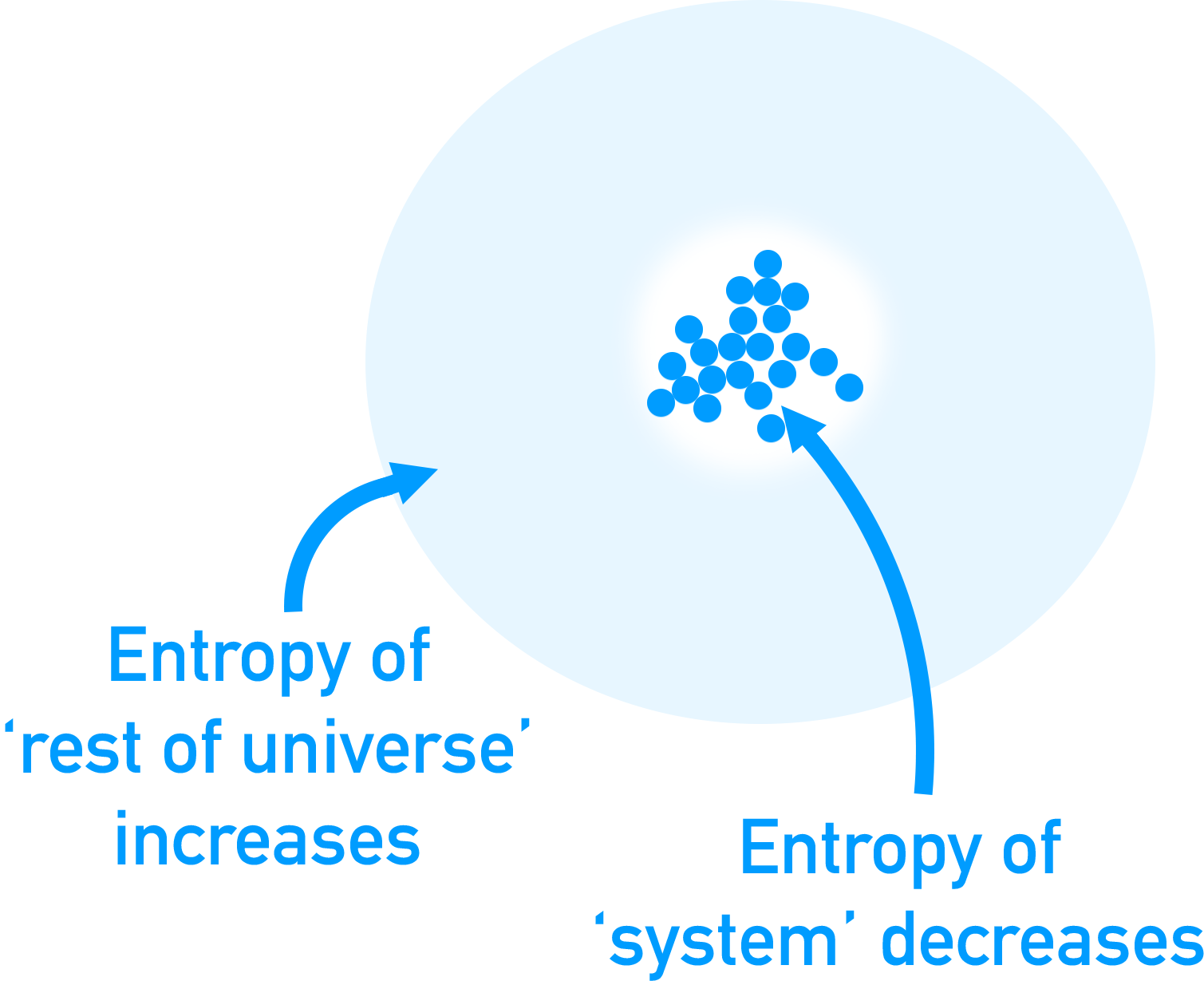The entropy of the system may have decreased here, but the energy required to pressurise the gas will have come from another process in which the entropy must have increased. As long as the entropy increase of the ‘rest of the universe’ is greater than the decrease in the entropy of the system, the reaction is ‘feasible’ (can happen).

This is why entropy is important to chemists, as it isn’t just enthalpy that changes in a chemical reaction, it is also entropy and both of these things have to be considered when looking at whether a reaction is able to occur.